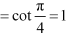### NCERT Solutions For Class 11 Math Chapter – 3 Exercise – 3.2

Find the values of other five trigonometric functions in Exercises 1 to 5.

1. cos x = -1/2, x lies in third quadrant.

Solution: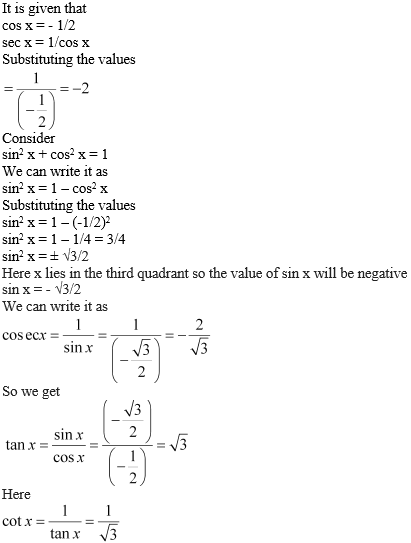2. sin x = 3/5, x lies in second quadrant.

Solution:

It is given that

sin x = 3/5

We can write it as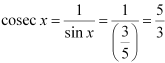We know that

sin2 x + cos2 x = 1

We can write it as

cos2 x = 1 – sin2 x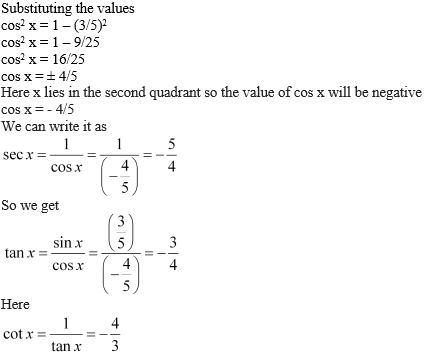3.

cot x = 3/4, x lies in third quadrant.

Solution:

It is given that

cot x = 3/4

We can write it as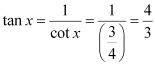We know that

1 + tan2 x = sec2 x

We can write it as

1 + (4/3)2 = sec2 x

Substituting the values

1 + 16/9 = sec2 x

cos2 x = 25/9

sec x = ± 5/3

Here x lies in the third quadrant so the value of sec x will be negative

sec x = – 5/3

We can write it as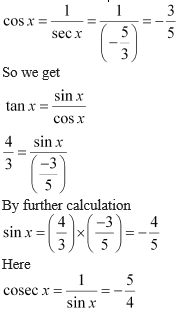4. sec x = 13/5, x lies in fourth quadrant.

Solution:

It is given that

sec x = 13/5

We can write it as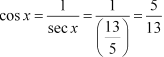We know that

sin2 x + cos2 x = 1

We can write it as

sin2 x = 1 – cos2 x

Substituting the values

sin2 x = 1 – (5/13)2

sin2 x = 1 – 25/169 = 144/169

sin2 x = ± 12/13

Here x lies in the fourth quadrant so the value of sin x will be negative

sin x = – 12/13

We can write it as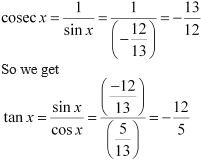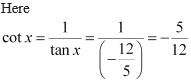5. tan x = -5/12, x lies in second quadrant.

Solution:

It is given that

tan x = – 5/12

We can write it as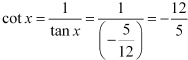We know that

1 + tan2 x = sec2 x

We can write it as

1 + (-5/12)2 = sec2 x

Substituting the values

1 + 25/144 = sec2 x

sec2 x = 169/144

sec x = ± 13/12

Here x lies in the second quadrant so the value of sec x will be negative

sec x = – 13/12

We can write it as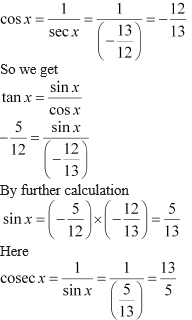Find the values of the trigonometric functions in Exercises 6 to 10.

6. sin 765°

Solution:

We know that values of sin x repeat after an interval of 2π or 360°

So we get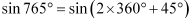By further calculation

= sin 45o

= 1/  2

7. cosec (–1410°)

Solution:

We know that values of cosec x repeat after an interval of 2π or 360°

So we get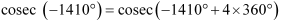By further calculation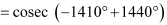= cosec 30o = 2

8.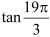Solution:

We know that values of tan x repeat after an interval of π or 180°

So we get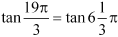By further calculation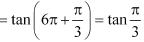We get

= tan 60o

3

9.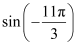Solution:

We know that values of sin x repeat after an interval of 2π or 360°

So we get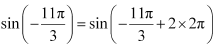By further calculation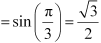10.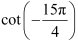Solution:

We know that values of tan x repeat after an interval of π or 180°

So we get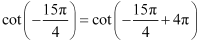By further calculation